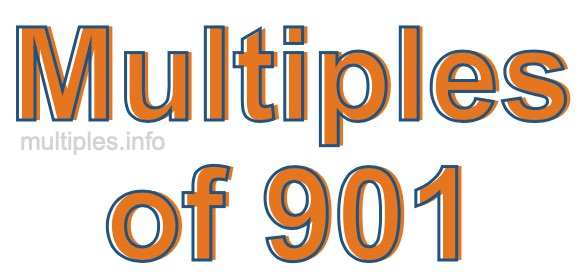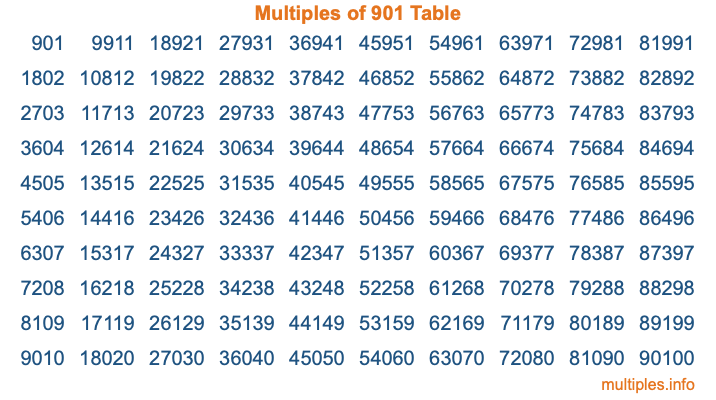Multiples of 901Welcome to the Multiples of 901 page. Here we will first teach you everything you will ever need to know about the multiples of 901, and then give you a study guide summary of everything we taught you to make sure you remember it all. Use this page to look up facts and learn information about the multiples of 901. This page will make you a multiples of nine hundred one expert!

Definition of Multiples of 901
Multiples of 901 are all the numbers that when divided by 901 equal an integer. Each of the multiples of 901 are called a multiple. A multiple of 901 is created by multiplying 901 by an integer.

Therefore, to create a list of multiples of 901, you start with 1 multiplied by 901, then 2 multiplied by 901, then 3 multiplied by 901, and so on for as long as you want. Thus, the list of the first five multiples of 901 is 901, 1802, 2703, 3604, and 4505. To see a larger list of multiples of 901, see the printable image of Multiples of 901 further down on this page. We also have a category where you can choose any nth multiple of 901.

Multiples of 901 Checker
The Multiples of 901 Checker below checks to see if any number of your choice is a multiple of 901. In other words, it checks to see if there is any number (integer) that when multiplied by 901 will equal your number. To do that, we divide your number by 901. If the the quotient is an integer, then your number is a multiple of 901.

Is  a multiple of 901?

Least Common Multiple of 901 and ...
A Least Common Multiple (LCM) is the lowest multiple that two or more numbers have in common. This is also called the smallest common multiple or lowest common multiple and is useful to know when you are adding our subtracting fractions. Enter one or more numbers below (901 is already entered) to find the LCM.

Check out our LCM Calculator if you need more details about the Least Common Multiple or if you need the LCM for different numbers for adding and subtraction fractions.

nth Multiple of 901
As we stated above, 901 is the first multiple of 901, 1802 is the second multiple of 901, 2703 is the third multiple of 901, and so on. Enter a number below to find the nth multiple of 901.

th multiple of 901

Multiples of 901 vs Factors of 901
901 is a multiple of 901 and a factor of 901, but that is where the similarities end. All postive multiples of 901 are 901 or greater than 901. All positive factors of 901 are 901 or less than 901.

Below is the beginning list of multiples of 901 and the factors of 901 so you can compare:

Multiples of 901: 901, 1802, 2703, 3604, 4505, etc.

Factors of 901: 1, 17, 53, 901

As you can see, the multiples of 901 are all the numbers that you can divide by 901 to get a whole number. The factors of 901, on the other hand, are all the whole numbers that you can multiply by another whole number to get 901.

It's also interesting to note that if a number (x) is a factor of 901, then 901 will also be a multiple of that number (x).

Multiples of 901 vs Divisors of 901
The divisors of 901 are all the integers that 901 can be divided by evenly. Below is a list of the divisors of 901.

Divisors of 901: 1, 17, 53, 901

The interesting thing to note here is that if you take any multiple of 901 and divide it by a divisor of 901, you will see that the quotient is an integer.

Multiples of 901 Table
Below is an image of the first 100 multiples of 901 in a table. The table is in chronological order, column by column. The first column has the first ten multiples of 901, the second column has the next ten multiples of 901, and so on.The Multiples of 901 Table is also referred to as the 901 Times Table or Times Table of 901. You are welcome to print out our table for your studies.

Negative Multiples of 901
Although not often discussed or needed in math, it is worth mentioning that you can make a list of negative multiples of 901 by multiplying 901 by -1, then by -2, then by -3, and so on, to get the following list of negative multiples of 901:

-901, -1802, -2703, -3604, -4505, etc.

Multiples of 901 Summary
Below is a summary of important Multiples of 901 facts that we have discussed on this page. To retain the knowledge on this page, we recommend that you read through the summary and explain to yourself or a study partner why they hold true.

There are an infinite number of multiples of 901.

A multiple of 901 divided by 901 will equal a whole number.

901 divided by a factor of 901 equals a divisor of 901.

The nth multiple of 901 is n times 901.

The largest factor of 901 is equal to the first positive multiple of 901.

901 is a multiple of every factor of 901.

901 is a multiple of 901.

A multiple of 901 divided by a divisor of 901 equals an integer.

901 divided by a divisor of 901 equals a factor of 901.

Any integer times 901 will equal a multiple of 901.

Multiples of a Number
Here you can get the multiples of another number, all with the same attention to detail as we did for multiples of 901 on this page.

Multiples of
Multiples of 902
Did you find our page about multiples of nine hundred one educational? Do you want more knowledge? Check out the multiples of the next number on our list!